# 5 Major Activation Function Used In Deep Learning And Why?

Activation Function is a major key to understand the working of neural network in deep learning, it is an activation function that determines whether the output of the set of a neuron or layer should be used further or not. For example, let us assume you like ice-cream and hates broccoli, so what happens in your brain when you see ice-cream? It stimulates or fires the neuron that suggests you eat it and in the case of broccoli, it would suggest you not to eat. In a broader perspective, this is how activation function in the neural network works.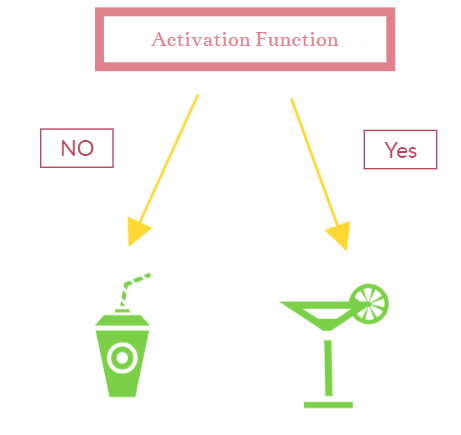## How does the Activation Function Work?

We have discussed why we should use the activation function or the intuition behind the activation function in a neural network, but to really be able to give a “thinking power” to a machine, shouldn’t it be a bit tough? Well, the answer is NO, it is not. So how does an activation function decides on its own, you would be thinking by implementing a threshold value to the output of a neuron may be a way for the activation function to decide which neurons to fire/activate and which do not, and yes, that is the perfect way to think about the decidability problem in activation function.

Let us take a neural network and analyze the working of an activation function in neural network-:Above is a pictorial representation of the working of the activation function, where P1 to Pn are inputs of the layer with weights w1 - wn associated with them, a bias ‘B’ is added to the activation function lastly. we could also formulate the above representation-: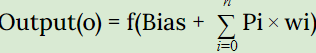Where f is an activation function.

What happens next? The same process is repeated in every layer of the deep neural network, so at last, we get our predicted value, also known as forward-propagation or feed-forward network.

However, in most cases, the difference between actual and predicted value would be high and we have to minimize this value with the help of a backpropagation algorithm in deep learning.

(Also read: Backpropagation algorithm in machine learning)

The importance of the activation function could be determined by the number of factors it improves in a deep neural network, the activation function just not helps in decision making but also adds the non-linearity to the model so that our model could learn complex patterns. Non-linearity in machine learning or neural network is a property with which our model is able to learn difficult functions.

Now we shall discuss the 5 major types of activation function used in neural network and other deep learning models, Activation Functions can be broadly described as two types-:

Linear Activation Function - That adds linearity to the model, linearity will be shown with the help of a straight line in general representation.

Non-Linear Activation Function - An activation function that adds non-linearity to a model so that it could learn more complex features from the network, Clearly, the non-linear activation function is way more powerful than the linear activation function, and henceforth, it is used more in the artificial neural network, autoencoders, and convolutional neural network. We would be covering one linear activation function example, and then we will dive into the 5 most used activation function in a neural network.

## Linear Activation Function

The linear activation function is not bounded in a certain range, i.e the range of linear activation function lies from -infinity to infinity, This is a very simple activation function without any threshold value and complex function, but this is not an advantage for our model, this is the weakness of linear activation function and the reason why it is almost not used at all.

Mathematically, the function could be defined as the sum of bias in a network and a weighted sum of input.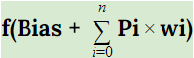Graphically, the linear activation function forms a straight line, that shows the linear nature of the function.

## 5 Most Used Activation Function Explained

We have now discussed the linear activation function and how it doesn’t help us with learning new and complex patterns, we shall now move to non-linear activation functions such as Sigmoid, Softmax, ReLu, Leaky ReLu, and TanH activation function

## Sigmoid Activation Function

Sigmoid is a non-linear activation function that means it will add non-linearity to the machine learning model, Unlike linear activation function, sigmoid is bounded within the range of 0 to 1, because the range is specified, therefore the output will be within this range only, so predicting capability of a model is seen to be significantly increased with the use of sigmoid activation function.

The more value is near to 1, the more likely it would be fired, and the more value is close to 0, the more likely case is that it wouldn’t be fired, therefore you could see how the threshold is working in the sigmoid activation function

Mathematically, if the input is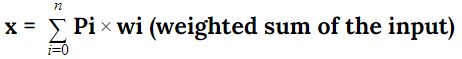Then the sigmoid function would be: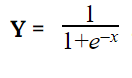, where ‘e’ is Euler’s number.

Graphically, it will be represented within the range of 0 to 1 and a non-linear graph is observed.## Softmax Activation Function

Softmax activation is another non-linear activation function that also has a probabilistic approach, so it is mainly used in the last layer, also known as the output layer, where we are predicting the values. Therefore, the inputs of the output layer are transformed into distinct values that are nothing but a probability distribution.

Let’s take the values to the input layer and add a softmax activation function to find the result, the result, as said, will be in the probability distribution and the sum of all values at as outcome will be 1.You can clearly observe the function of the softmax activation function with the help of the above visualization.

Mathematically, the formula for softmax activation function is represented as-: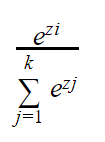This formula shows an exponential function that is associated with every element in the output layer divided by the sum of the exponential functions.

Graphically, the distribution will be directly proportional to the output layer values.## TanH Activation Function

TanH is one of the most used activation function and in the words of Andrew Ng, TanH is better than the sigmoid activation function, although it depends on many factors, one such factor is on which interval we want the output of the activation function, the interval or range of the TanH activation function is from -1 to 1, This range is the main reason behind the argument that for efficient backpropagation, TanH is a better choice than sigmoid as the average output will lie within the range of 0 which is better than the 0.5 average of sigmoid and will increase the next layer learning capability.

Mathematically, it is derived as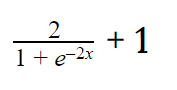Graphically, the TanH activation function also produces an ‘S’ shaped representation.## ReLu Activation Function

Arguably, the most important activation function is the ReLu activation function as it has an edge over other activation functions and is widely preferred by AI educators and researchers. To understand why ReLu is better than TanH and sigmoid, we have to understand the saturation problem in both sigmoid and TanH.

## Problems With Sigmoid And TanH Activation Function

The saturation problem we talked about means that for the greater values of the input, TanH and sigmoid will convert the value to ‘1’, and for smaller value, TanH and sigmoid will covert the value to ‘-1’ and ‘0’ respectively, therefore when saturation problem exist, the model would not be able to extract the useful information as data is being sensitive at certain average values like ‘0’ and ‘0.5’ in the cases of TanH and sigmoid respectively.

## Why Relu Is Preferred over TanH and Sigmoid?

The reLu activation function eliminates the problem of saturation from the model, the range of the Rectified linear unit(ReLu) is in the range of ‘0’ to infinity, while the input values are negative, it returns 0, and for the positive input value, it returns that value itself.

Mathematically, the formula goes from

R(z) = max( 0, z)

Graphically, the ReLu activation function is represented as below:-Relu is the most used activation function in deep learning and is used in almost every neural network by default. Although there is one disadvantage of the ReLu activation function that by converting all the negative values to 0, it becomes slightly difficult to train the model as for 0 value, our model will learn nothing and eventually there might be the case where it stops learning, popularly known as ‘Dying Relu’ problem.

## Leaky ReLu Activation Function

To save the dying problem of the Relu activation function, we use the Leaky ReLu activation function, this function multiplies some value to the negative input values so that the learning doesn’t stop.

For negative input x, x0.01 value may be added

Graphically, we could see the difference in the negative portion when compared to the ReLu activation function.## Conclusion

Over the years, many activation functions arrived and showed their potential, for example, sigmoid and tanH were highly used in the 1990s before the arrival of ReLu and Leaky ReLu, Right now, ReLu and Leaky ReLu are considered to be the most powerful activation function.### tanesh

Founder Of Aipoint, A very creative machine learning researcher that loves playing with the data.